# Exercises

## Information Theory, Inference and Learning Algorithms

### 6.14

Since there is no covariance between the different dimensions, i.e. the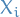are independent of all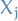with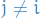, we know that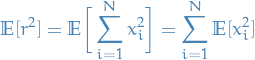where each of the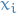are following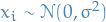, hence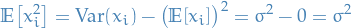Hence,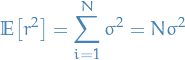The variance is then given by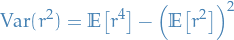where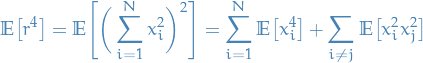But since there is no covariance between the different, the second sum vanishes, and since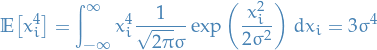(which we knew from the hint 6.14 in the book). Hence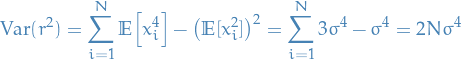This all means that for large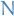, we will have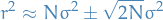And since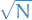will be neglible for large, compared to(of course assuming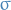is finite), then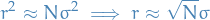as watned. The "thickness" will simply be the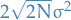, i.e. twice the variance of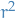.

Either by:

• Computing andimensional integral :)
• Empirically looking at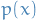for some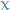and making use of the symmetry of the Gaussian to infer that all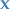with same radius have the same probability, and that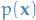decreases whenmoves away (in whatever "direction" / dimension) from the mean